# Can ultrasound be used to stimulate nerve tissue?

## Abstract

### Background

The stimulation of nerve or cortical tissue by magnetic induction is a relatively new tool for the non-invasive study of the brain and nervous system. Transcranial magnetic stimulation (TMS), for example, has been used for the functional mapping of the motor cortex and may have potential for treating a variety of brain disorders.

### Methods and Results

A new method of stimulating active tissue is proposed by propagating ultrasound in the presence of a magnetic field. Since tissue is conductive, particle motion created by an ultrasonic wave will induce an electric current density generated by Lorentz forces. An analytical derivation is given for the electric field distribution induced by a collimated ultrasonic beam. An example shows that peak electric fields of up to 8 V/m appear to be achievable at the upper range of diagnostic intensities. This field strength is about an order of magnitude lower than fields typically associated with TMS; however, the electric field gradients induced by ultrasound can be quite high (about 60 kV/m2 at 4 MHz), which theoretically play a more important role in activation than the field magnitude. The latter value is comparable to TMS-induced gradients.

### Conclusion

The proposed method could be used to locally stimulate active tissue by inducing an electric field in regions where the ultrasound is focused. Potential advantages of this method compared to TMS is that stimulation of cortical tissue could be highly localized as well as achieved at greater depths in the brain than is currently possible with TMS.

## Background

Much has been written in the last decade about the potential of transcranial magnetic stimulation (TMS) as a new diagnostic and therapeutic tool in clinical neurophysiology . TMS is a painless and non-invasive technique for stimulating cortical tissue by means of magnetic induction. TMS has been used to map the motor cortex by recording evoked responses, and appears to show potential for treating certain brain disorders, from Parkinson's disease to depression. A disadvantage of TMS, however, is its inability to localize the site of excitation in the brain to a volume less than several tens of cubic centimeters at best. This is because the spatial distribution of the magnetic fields cannot be effectively concentrated or focused. In fact, because the fields obey Laplace's equation, it is impossible to create local maxima in the field intensity no matter what the configuration of source coils; in a homogeneous conducting medium, the field strength will always be largest at the surface and fall off monotonically at greater depths.

In this paper, we propose a different method of stimulating cortical tissue, which, in principle, could permit much greater localization of the site of excitation as well as deeper penetration into the brain. The idea is to induce electrical currents by propagating an ultrasonic wave in the presence of a strong DC magnetic field. The currents are generated by Lorentz forces on moving ions produced by the oscillating sound wave.1

We present here an analysis of the distribution of the electric field induced by an ideally collimated ultrasonic beam in a homogeneous conducting medium. The analysis allows us to calculate the magnitude of the electric field strengths that can be expected, and shows that the highest practical fields that can be induced by ultrasound, up to about 8 V/m, are about an order of magnitude lower than those associated with TMS. Such fields have been reported in the literature to range between about 10 and 100 V/m . We note, however, that the spatial and temporal character of the ultrasonically-induced fields are quite different from those induced by magnetic induction. One such difference is that the spatial variations of the ultrasonically-induced fields are much more rapid than the fields associated with TMS since, in the former case, the fields change on the scale of the ultrasonic wavelength (e.g., less than a millimeter). Thus, the magnitude of the gradients of the ultrasonically-induced electric fields can be comparable to those associated with TMS, depending on the ultrasonic frequency (e.g., at 4 MHz, a gradient of about 60 kV/m2 is attainable). This may be significant since activation models predict that the gradient of the field, rather than its strength, is primarily responsible for neural stimulation [6, 7]. However, researchers have also speculated that field gradients induced by cellular-scale inhomogeneities in the tissue conductivity may play a more important role in neural stimulation than the spatial variations of the impressed fields . The latter question is important in deciding whether the potentially large impressed field gradients induced by ultrasound are truly an advantage in effecting stimulation.2

A second difference between ultrasonic stimulation and TMS that may have some practical significance for exciting neural tissue is related to our greater freedom in controlling the time dependence of an ultrasonic pulse compared to a TMS pulse. We show below that the temporal dependence of the electric field (and the induced current density) follows that of the ultrasonic waveform. Although the duration of a TMS pulse is typically on the order of a few tenths of a millisecond and the ultrasonic variation is on the order of microseconds or less (roughly the reciprocal of the ultrasonic frequency), considerable control over the shape of the ultrasonic waveform is in principle possible. For example, one can transmit an ultrasonic pulse train at essentially any repetition frequency or modulate a continuous ultrasonic wave in a variety of ways.

For neural excitation to take place, the magnitude of the induced electric field (or its gradient) must exceed an activation threshold. It is generally believed that excitation that is oscillatory in time makes this process more difficult. For example, simulations carried out by Reilly suggest that, using a sinusoidal stimulus, the activation threshold increases monotonically with frequency beyond a few kHz . However, Reilly also reports that a series of rapid pulses, where the repetition period is short compared to the depolarization time constant (the latter being a few tenths of a millisecond), is a much more effective stimulus than a pure sinusoid. Reilly describes this as the "integration effect of multiple pulses." If the individual pulses are monophasic, the activation threshold is considerably reduced compared to a single monophasic pulse. For example, Reilly reports that the integration effect of a series of rapid pulses, each 10 microsec in duration with a repetition rate of 50 kHz, reduces the activation threshold by a factor of 5 relative to a single 10 microsec pulse.

A key question is whether a series of pulses of this kind can be generated ultrasonically. Certainly, the 50 kHz rate is feasible. A second question is whether one can create an ultrasonic pulse that is approximately monophasic. With some ingenuity, the answer appears to be yes. For example, although an ultrasonic pulse must have a zero DC component, the pulse need not be symmetric; that is, the positive-going excursion of an ultrasonic pulse can, under certain conditions, significantly exceed the negative-going excursion, which would approximate a "monophasic" waveform. A simple example of the latter can be achieved with the aid of an ultrasonic transducer that is non-symmetric, e.g., a transducer whose length is much greater than its width. In this case, there is a large positive excursion followed by a much longer, but weaker, negative excursion (an ultrasonic "wake"). Another way to alter the shape of an ultrasonic pulse is by transmitting it through a dispersive medium that acts as an ultrasonic filter, or by using multi-layered transducers.

Although, in our analysis, we shall for simplicity assume a static magnetic field, an alternative approach is to use an optimally-designed magnetic pulse produced by a conventional TMS coil to bring the neural tissue close to its activation threshold, but not exceeding it. TMS coils create fields, although transient, in the range of 1 T to 4 T. An ultrasonic pulse (or series of pulses), if properly timed, could then push the membrane potential past threshold. In this way, the locus of activation would still be determined by the ultrasound, although deep stimulation might still be difficult because the field intensity produced by a TMS coil falls off rapidly with depth (a problem avoided by using a static field). It is worth noting that the idea of using a conditioning pulse of this kind was suggested by Reilly , but in a somewhat different context. Reilly proposed using two coils, one that produces a "conditioning pulse" (Reilly's phrase) to achieve 75% of the excitation threshold; then a second nearby coil, excited by a sinusoidal waveform (of frequency 80 kHz in Reilly's example), would push the membrane potential beyond threshold. His idea was that the combination of coils is more effective in achieving greater "focality," or localization, of the stimulus than a single coil. Using a mathematical model of neural activation, Reilly predicts that this combination of signals, the conditioning pulse plus the 80 kHz sine wave, will achieve activation. One could speculate that, instead of the 80 kHz sinusoidal signal, the conditioning pulse could be accompanied by a series of ultrasonic pulses at a rate of 80 kHz (or whatever rate is determined to be optimal). It may turn out that these questions can only be settled by experiment, or by sophisticated modeling of the neuron in the presence of a realistic ultrasonic wave.

## Method

### Concept

Localized electric currents can be induced in tissue by propagating ultrasound in the presence of a magnetic field. Consider, for example, an ultrasonic pulse propagating in an electrolytic fluid (such as soft tissue) in the presence of a static magnetic field oriented in a direction perpendicular to the propagation path. The longitudinal particle motion due to the ultrasonic wave moves the ions back and forth through the magnetic field; this results in Lorentz forces on the ions that give rise to an electric current density that oscillates at the ultrasonic frequency. This idea was used as the basis of "Hall effect imaging" proposed by Wen  in which Lorentz forces are employed to image the electrical conductivity of tissue. Montalibet et al. also noted that propagating ultrasound in the presence of a magnetic field will generate electric currents in tissue . They also proposed using this effect to measure the electrical conductivity of tissue.

Here we propose a new application: localized stimulation of active (nerve or cortical) tissue by ultrasonically-induced electric fields. Our objective in this paper is to compute the magnitude and the spatial distribution of the these fields. We shall show that analytical solutions for the field distribution can be derived for an ideally collimated ultrasonic beam.

### Theory

Consider an ion in a conductive medium with charge q. The longitudinal particle motion of an ultrasonic wave will cause the ion to oscillate back and forth in the medium with velocity v. In the presence of a constant magnetic field, B 0, the ion is subjected to the Lorentz force

F = q v × B 0.     (1)

This produces an electric current density given by

J 0 = (n + u + + n - u -)F,     (2)

where u + and u - are the mobilities of the positive and negative ions (assumed to have charges of q and -q, respectively), and n + and n - are their concentrations. Combining (1) and (2) gives

J 0 = q(n + u + + n - u -)v × B 0.     (3)

But the electrical conductivity, σ, of the medium is given by

σ = q(n + u + + n - u -),     (4)

so that

J 0 = σv × B 0.     (5)

A typical value of the conductivity of tissue is 0.5 Siemens/m. In the most general case, the conductivity σ may be regarded as a complex quantity to account for polarization (or displacement) currents at higher frequencies. From (5), the equivalent electric field is

E 0 = v × B 0.     (6)

The field, E 0, and the current density, J 0, oscillate at the ultrasonic frequency in a direction mutually perpendicular to the propagation path (the direction v) and the magnetic field B 0.

For convenience, we assume harmonic excitation of the ultrasonic wave of the form exp(-i ωt). Since all our equations are linear, an arbitrary time dependence can be treated by Fourier synthesis. In the following, the time dependence of all quantities, both ultrasonic and electromagnetic, will be exp(-i ωt), so this factor is dropped. The ultrasonically-induced current density J 0 can be regarded as an impressed current density that gives rise to scattered (or secondary) electric and magnetic fields E s and B s that obey the Maxwell's equation× B s = μ0σE s + μ0 J 0,     (7)

where μ0 is the free-space magnetic permeability. Dielectric properties of tissue can be accounted for by replacing σ everywhere with σ - i ωε where ε is the tissue permittivity. At a frequency of about 1 MHz and using typical values for tissue, the quantity ωε will be one to two orders of magnitude less than σ.

Substituting (5) and (6) into (7), we have× B s = μ0σ(E s + E 0).     (8)

In this equation, the impressed electric field is E 0, given by (6), and E s is the induced electric field whose source is the Lorentz-induced current density (5). The total electric field is the sum

E = E s + E 0,     (9)

and the total current density is

J = σE s + J 0 = σE.     (10)

Here our objective is to compute the induced field, E s , to be substituted into (9) to obtain the total field, E.

To solve this problem, consider a collimated ultrasonic beam propagating in the z-direction, assumed here to have axial symmetry with a radial profile given by p(r), where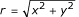. The particle velocity is then given by: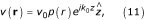where v 0 is the peak particle velocity,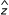is the z-directed unit vector along the beam axis, k 0 = ω/c 0, and c 0 is the ultrasonic wave speed. Although this beam is idealized (e.g., it neglects spreading), (11) is a reasonable approximation to a focused ultrasonic beam in the region of its focus. In the presence of a magnetic field B 0 = B 0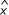, the resultant Lorentz-induced current density is predicted by (5) to be:

J 0(r) = B 0 v 0σp(r)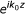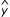.     (12)

When this current density is substituted into (7), one can solve for the induced electric field, E s , using standard techniques. As shown in the appendix, the components of E s in cylindrical coordinates are found to be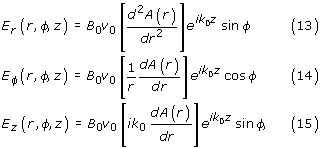where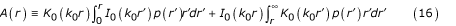and I 0(·) and K 0(·) are modified Bessel functions of the first and second kind. At this point, for an arbitrary beam profile p(r), one must resort to a numerical integration of (16). However, the integrals can be evaluated analytically for the special case of an ideally collimated ultrasonic beam whose radial profile is defined bywhere a is the beam radius. Substituting (17) into (16), we find :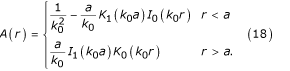Inserting this result into (13)-(15), we obtain the components of the induced electric field E s , as follows. Inside the beam (r <a), we have

E r (r,φ,z) = B 0 v 0 P r (r)sin φ     (19)

E φ(r,φ,z) = B 0 v 0 P φ(r)cos φ     (20)

E z (r,φ,z) = B 0 v 0 P z (r)sin φ     (21)

where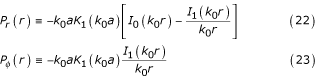P z (r) ≡ -ik 0 a K 1(k 0 a) I 1(k 0 r),     (24)

and outside the beam (r >a),

E r (r,φ,z) = B 0 v 0 Q r (r)sin φ     (25)

E φ(r,φ,z) = B 0 v 0 Q φ(r)cos φ     (26)

E z (r,φ,z) = B 0 v 0 Q z (r)sin φ     (27)

where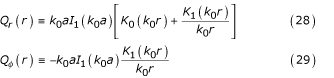Q z (r) ≡ -ik 0 a I 1(k 0 a) K 1(k 0 r).     (30)

On the beam boundary (r = a), the tangential components of the electric field are continuous as expected; that is, P φ (a) = Q φ (a) and P z (a) = Q z (a). This can be checked by setting r = a in the above equations and using the identity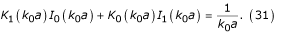By comparing P r (a) and Q r (a), however, we see that the radial component of the field is discontinuous on the boundary, indicating charge accumulation there. This charge, of course, oscillates at the ultrasonic frequency. The radial electric field discontinuity is seen to be

ΔE = B 0 v 0[Q r (a) - P r (a)] sin φ= B 0 v 0 sin φ,     (32)

where (31) was used in the last step. This result can be employed to compute the surface charge density, ρ s , on the beam boundary by means of the relation ρ s = -εΔE, where ε is the tissue dielectric constant.

It can be shown that the radially-dependent terms (22)-(24) and (28)-(30) achieve their maximum values near r = a, that is, near the beam boundary. In this region, the terms with k 0 r in the denominator can be neglected compared to the other terms. Thus, the dominate terms when r is near a are given by:

for r <a,

P r (r) = -B 0 v 0 k 0 a K 1(k 0 a) I 0(k 0 r)     (33)

P φ(r) = 0     (34)

P z (r) = -iB 0 v 0 k 0 a K 1(k 0 a) I 1(k 0 r),     (35)

for r >a,

Q r (r) = B 0 v 0 k 0 a I 1(k 0 a) K 0(k 0 r)     (36)

Q φ(r) = 0     (37)

Q z (r) = -iB 0 v 0 k 0 a I 1(k 0 a) K 1(k 0 r).     (38)

Let us evaluate these functions on the beam boundary (r = a). The arguments of the Bessel functions are k 0 a = 2πa/λ, so that, for a greater than a few wavelengths, we can use the large argument asymptotic approximations :which giveswhere we have defined E 0B 0 v 0 as the magnitude of the impressed electric field, as seen from (6).

Several comments about this solution are worth noting. First, for a beam with a perfectly (and unrealistically) sharp boundary at r = a, the radial component of the electric-field gradient is infinite on the boundary. This is a mathematical artifact of our solution since the electric field is discontinuous at r = a. For a real beam, the transition is much more gradual (on the order of an ultrasonic wavelength or more), and charge distribution on the beam boundary will be distributed over this transition region. A second point is that a numerical integration of (16) for a more realistic beam profile, p(r), that falls off more smoothly than (17), such as a Gaussian profile, results in an electric field smaller in magnitude at the beam boundary than that given by (39) and (40). Thus, the above analytical solution provides a useful, but very qualitative, picture of the field behavior at the beam boundary. It can also be seen from the expressions (22)-(24) that the induced field is near zero at the center of the beam (r = 0). In fact, (24) predicts that the z-component of the induced field is exactly zero at r = 0 and that the other components are very small compared to E 0 at r = 0. The latter statements also hold true for a Gaussian beam profile, as a numerical integration shows. This is a consequence of the fact that E s arises essentially due to charge accumulation in the vicinity of the beam boundary. Thus, on the beam axis, the total electric field, E 0 + E s , is close to the Lorentz induced field E 0.

We next consider the gradient of the electric field. For activation, we are interested in the rate of change of the component of the electric field along the direction of the axon. If we regard the significant field as E 0 only, then we note that this field is perpendicular to the beam axis, while the largest component of the gradient points along the beam axis and is given by the derivative of E 0 with respect to z. If the axon is oriented at an angle α with respect to the beam direction, then the component of the electric field along the direction of the axon is E 0 sin α. Now the rate of change of this component along the axon direction is the derivative of E 0 sin α with respect to the distance measured along the axon, which is (ik 0 cos α)E 0 sin α. The magnitude of this quantity is largest for α = ± 45° and equals k 0 E 0/2 = k 0 B 0 v 0/2. In the next section, we estimate the size of E 0 and the gradient k 0 E 0/2 for a practical example.

## Results and Discussion

Here we estimate the peak electric field that can be safely induced using ultrasound at the upper range of diagnostic intensities. A key parameter in computing the field strength is the particle velocity generated by the ultrasonic wave. The peak particle velocity, v 0, can be related to the peak pressure, p 0, of the wave using the relationwhere ρ is the tissue density and c 0 is the sound speed. Measurements performed by Duck  using two modern commercial ultrasonographic scanners have demonstrated instantaneous peak pressures in excess of 4 MPa at the focal point of the transducer. 3 (Although this pressure translates into an intensity that considerably exceeds the temporally-averaged FDA limit of 0.7 W/cm2 for diagnostic systems, the instantaneous intensities are generally far higher than the latter value.)

Using the values of p and c 0 for water, the above formula gives a peak particle velocity, v 0, of 2.67 m/s. To estimate the magnitude of E 0 for a typical set of parameters, we assume a magnetic field strength of 1.5 Tesla, which is typical of whole body MRI magnets. This gives E 0 = 4 V/m. The electric field strength can, of course, be enhanced by increasing the magnetic field B 0. Although 1.5 T is a typical MRI field strength, 3 T systems have recently become available. This field strength would double the peak electric field to E 0 = 8 V/m. It may be worth noting that a high-field magnet with a smaller bore designed to accommodate only the head would be much less costly than the whole-body machines with much larger bore diameters. In general, smaller bore magnets are also more suitable for high-field applications. Finally, the very high magnetic field homogeneity needed for imaging is not required in this application, which would further reduce costs.

We next estimate the maximum gradient of the electric field, given earlier by k 0 E 0/2. The particle velocity quoted above was measured for an instrument operating at an ultrasonic frequency of 4 MHz . Using E 0 = 8 V/m, we obtain an electric field gradient of k 0 E 0/2 = 67 kV/m2 at this frequency. This is of the same order of magnitude of the activation thresholds computed by Hsu and Durand .

## Conclusion

Although the strength of the ultrasonically-induced electric fields is about an order of magnitude below that of TMS generated fields, there are differences in the spatial and temporal character of these fields that may have some practical consequences. The first point is that the ultrasonically-induced electric field gradients are comparable to typical TMS gradients, because the ultrasonically-induced fields pass from maximum to minimum in a much shorter distance, i.e., approximately half the ultrasonic wavelength (a fraction of a millimeter). A second point is that one can exercise greater control over the temporal shape of the ultrasonically-induced electric fields compared to TMS-induced fields. For example, the excitation can be oscillatory ("polyphasic") or not (approximately "monophasic," with a sharp upgoing excursion followed by a weaker, but longer negative going excursion). Pulses can be applied in very rapid sequence, or a continuous wave can be modulated in a variety of ways.

We also mentioned the possibility of using a conventional TMS coil to generate a strong, but transient, magnetic field that could be applied during the transmission of the ultrasound. That is, the function of the TMS pulse would be to bring the membrane potential near threshold, but not to exceed it. The ultrasonic pulse (or pulses) would then push the potential past threshold. One could speculate that near threshold, where the membrane nonlinearity is strong, a sinusoidal stimulus may be effective in achieving activation due to some partial rectification of the sinusoid .

We conclude by noting that the same technique could be used in principle to stimulate any type of excitable tissue, not just cortical tissue, including, for example, peripheral nerve tissue. Finally, it may turn out that the ultrasonically-induced fields are simply too weak for effective stimulation, although this remains to be established. Answers to these questions may have to await experimental work or further modeling of the electrophysiology of neural activation.

## Appendix: Derivation of the Induced Electric Field

Here we wish to solve the Maxwell equation× B s = μ0σE s + μ0 J 0     (A1)

for E s where J 0 is given by (12). The problem is most easily solved by writing the magnetic and electric fields, B s and E s , in terms of vector and scalar potentials A and Φ:

B s =× A     (A2)

E s = i ωA -Φ.     (A3)

Substituting (A2) and (A3) into (A1) and using the identity×× A =(· A) -2 A, results in(· A) -2 A = i ωμ0σA - μ0σΦ + μ0 J 0.     (A4)

It is convenient to use the Lorentz gauge, defined bywhich results in two uncoupled equations for the potentials A and Φ. Substituting (A5) into (A4), we obtain2 A + γ2 A = -μ0 J 0,     (A6)

where γ2 = i ωμ0σ, and (A3) becomes upon substituting (A5)The solution to the vector Helmholtz equation (A6) is given by the following volume integral:where= (1 + i)/δ andis the electromagnetic skin depth in the tissue. The exponential term in the integrand of (A8) can be replaced by unity when δ |r - r'|. For example, at a frequency of 1 MHz and conductivity of 0.5 S/m, we find δ = 71 cm. Thus, to an excellent approximation, (A8) may be replaced by:We now argue that the first term on the right-hand side of (A7) is much smaller than the second term. In fact, the magnitude of the first term is smaller than the second term by a factor of the order (λ/δ)2, where λ is the ultrasonic wavelength and δ is the electromagnetic skin depth in tissue. This can be demonstrated rigorously for the case of the ideally-collimated ultrasonic beam treated in the main text, but a plausibility argument can be given as follows. The term(·A) in (A7) indicates terms that are second derivatives of the spatial dependence of the ultrasonic beam, which will be of the orderA. Then the ratio of the first term in (A7) to the second term is ωμ0σ/= λ2/(2π2δ2), whereis the skin depth and λ = 2π/k 0 is the ultrasonic wavelength. At an ultrasonic frequency of 1 MHz, this ratio is on the order of 10-6. Thus, neglecting the first term in (A7), we haveNext, substituting (12) into (A9), we see that the only non-zero component of A is its y-component, i.e., A = A y, so that (A9) becomes on writing the variables in cylindrical coordinates:We now employ the expansion of 1/|r - r'| in cylindrical coordinates :where I m (·) and K m (·) are the modified Bessel functions of the first and second kind of order m. In (A12), r <is the smaller of r and r', and r >is the larger. Substituting (A12) into (A11), interchanging orders of integration, and performing the φ' integral, only the m = 0 term survives, givingNext the integration with respect to z' is performed by noting thatwhere δ(·) is the Dirac delta function. Substituting this into (A13) and integrating with respect to k gives:For brevity, we write this as

A y (r, z) = B 0 v 0μ0σA(r),     (A16)

whereNoting that A =A y , we haveFinally, substituting this into (A10) and noting that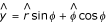, we obtain (13)-(15).

## Footnotes

1Fry appears to have been the first person to propose using ultrasound to stimulate the brain, although by means of a very different mechanism . His idea was to transmit ultrasound in the presence of an oscillating electric field of the same frequency. The periodic variation of the electrical conductivity of the tissue created by the slight variation in thermal expansion arising from the oscillating sound wave would, in principle, produce a partial rectification of the applied field, resulting in a small amount of unidirectional electric current. The resulting unidirectional charge transfer, Fry speculated, could stimulate neural tissue. However, to succeed, the method requires extremely intense ultrasonic pulses (from 10 to 50 kW/cm2) and a very strong electric field (producing current densities on the order of amps/cm2). As far as this author is aware, Fry's method has not been tried.

2A great deal has been written on the subject of what temporal and spatial features of the stimulating fields are responsible for activation. Early work by Rattay suggested that the "activation function," essentially the gradient of the electric field along the axis of the axon, is a key contributor . Other researchers pointed out that this gradient is determined not only by the variations in the impressed field, but also by conductance variations in the axon due to bends, terminations and other departures from a straight line that may perturb the local boundary conditions. Also, the shape and duration of the stimulating pulse play an important role. It has been noted that the time and space dependence of the activating function mutually interact in a way that may make the effects of each difficult to treat independently [13, 14].

3Note that we are assuming that the maximum intensity occurs at the focal point of the transducer. This is generally true if the ultrasound is sufficiently focused, but may not be true for a weakly focused transducer when the beam passes through an intervening medium that is very attenuating (such as the skull).

## References

1. Paulus W, Ed: Transcranial magnetic stimulation: proceedings of the International Symposium on Transcranial Magnetic Stimulation: 1998 Sept 30-Oct 4 Gottingen. New York, Elsevier 1999.

2. Pascual-Leone A, Ed: Handbook of transcranial magnetic stimulation London, Oxford University Press 2002.

3. Hallett M: Transcranial magnetic stimulation and the human brain. Nature 2000, 406: 147–150. 10.1038/35018000

4. Fry JF: Electrical simulation of the brain localized without probes – Theoretical analysis of a proposed method. J Acoust Soc Amer 1968, 44: 919–931.

5. Roth BJ, Saypol JM, Hallett M, Cohen LG: A theoretical calculation of the electric field induced in the cortex during magnetic induction. Electroencephalography Clin Neurophys 1991, 81: 47–56. 10.1016/0168-5597(91)90103-5

6. Rattay F: Analysis of models for external stimulation of axons. IEEE Trans Biomed Eng 1986, 33: 974–977.

7. Warman EN, Grill WM, Durand D: Modeling the effects of electric fields on nerve fibers: determination of excitation thresholds. IEEE Trans Biomed Eng 1992, 39: 1244–1254. 10.1109/10.184700

8. Nagarajan SS, Durand DM, Warman EN: Effects of induced electric fields on finite neuronal structures: a simulation study. IEEE Trans Biomed Eng 1993, 40: 1175–1187. 10.1109/10.245636

9. Maccabee PJ, Amassian VE, Eberle LP, Cracco RQ: Magnetic coil stimulation of straight and bent amphibian and mammalian peripheral nerve in vitro: locus of excitation. J Physiology 1993, 460: 201–219.

10. Ruohonen J, Ravazzani P, Grandori F: An analytical model to predict the electric field and excitation zones due to magnetic stimulation of peripheral nerves. IEEE Trans Biomed Eng 1995, 42: 158–161. 10.1109/10.341828

11. Sobie EA, Susil RC, Tung L: A generalized activating function for predicting virtual electrodes in cardiac tissue. Biophysical J 1997, 73: 1410–1423.

12. Kamitani Y, Bhalodia VM, Kubuta Y, Shimojo S: A model of magnetic stimulations of neocortical neurons. Neurocomputing 2001, 38–40: 697–703. 10.1016/S0925-2312(01)00447-7

13. Plonsey R, Barr RC: Electric field stimulation of excitable tissue. IEEE Trans Biomed Eng 1995, 42: 329–336. 10.1109/10.376126

14. Barr RC, Plonsey R: Threshold variability in fibers with field stimulation of excitable membranes. IEEE Trans Biomed Eng 1995, 42: 1185–1191. 10.1109/10.476125

15. Reilly JP: Peripheral nerve stimulation by induced electric currents: exposure to time-varying magnetic fields. Med Biol Eng Comp 1989, 27: 101–110.

16. Reilly JP: Applied Bioelectricity. New York, Springer 1998, 404.

17. Wen H, Shah J, Balaban RS: Hall effect imaging. IEEE Trans Biomed Eng 1998, 45: 119–124. 10.1109/10.650364

18. Montalibet A, Jossinet J, Matias A, Cathignol D: Electric current generated by ultrasonically induced Lorentz force in biological media. Med Biol Eng Comp 2001, 39: 15–20.

19. Abramowitz M, Stegun IA, Eds: Handbook of Mathematical Functions. New York, Dover Publications 1965, 484.

20. Abramowitz M, Stegun IA, Eds: Handbook of Mathematical Functions. New York, Dover Publications 1965, 375.

21. Abramowitz M, Stegun IA, Eds: Handbook of Mathematical Functions. New York, Dover Publications 1965, 377–378.

22. Duck FA: Estimation in situ exposure in the presence of acoustic nonlinearity. J Ultrasound Med 1999, 18: 43–53.

23. Hsu KH, Durand DM: Prediction of neural excitation during magnetic stimulation using passive cable models. IEEE trans Biomed Eng 2000, 47: 463–471. 10.1109/10.828146

24. Reilly JP: Applied Bioelectricity. New York, Springer 1998, Ch. 4.

25. Jackson JD: Classical Electrodynamics. New York, Wiley 2 Edition 1975, 220.

26. Jackson JD: Classical Electrodynamics. New York, Wiley 2 Edition 1975, 118.

## Author information

Authors

### Corresponding author

Correspondence to Stephen J Norton.

## Rights and permissions

Reprints and Permissions

Norton, S.J. Can ultrasound be used to stimulate nerve tissue?. BioMed Eng OnLine 2, 6 (2003). https://doi.org/10.1186/1475-925X-2-6

• Accepted:

• Published:

• DOI: https://doi.org/10.1186/1475-925X-2-6

### Keywords

• Transcranial Magnetic Stimulation
• Ultrasonic Wave
• Lorentz Force